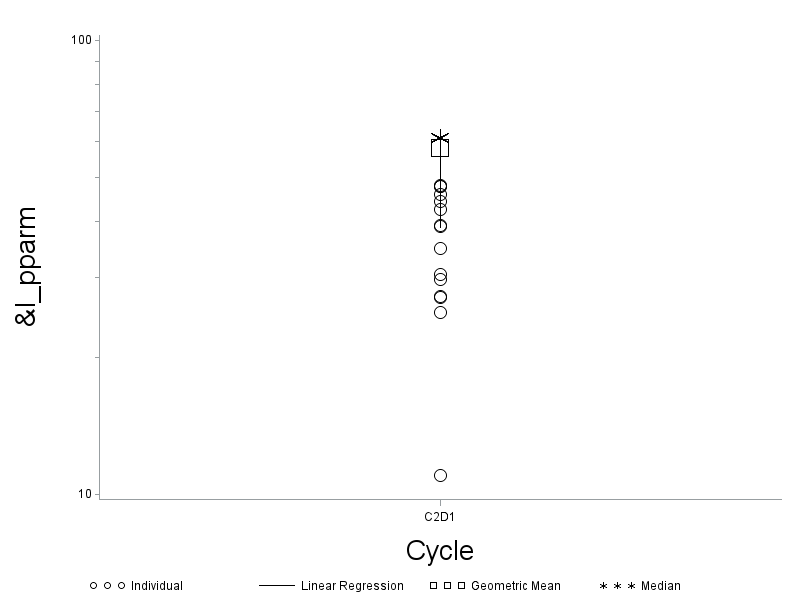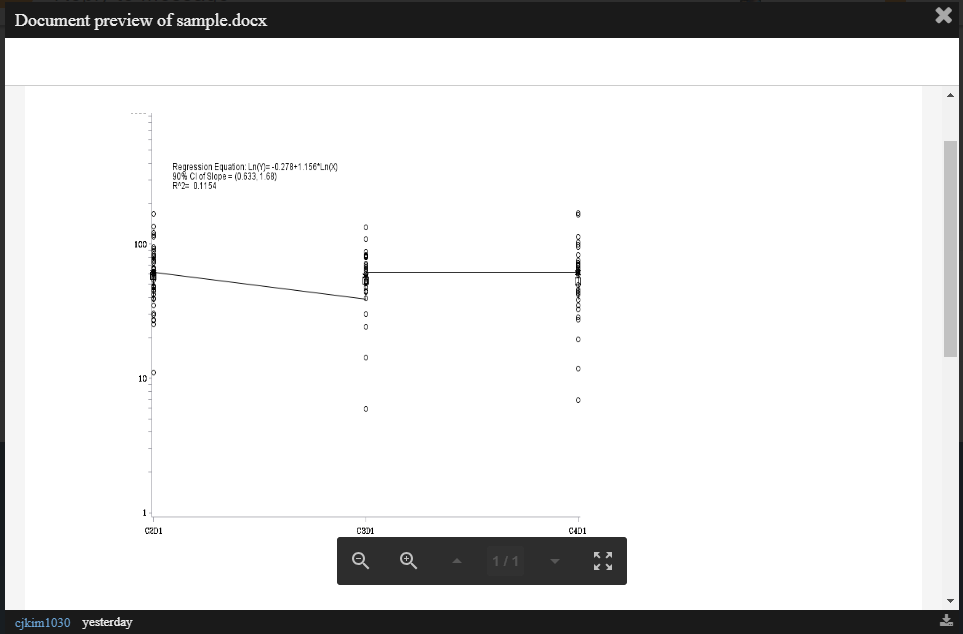## Regression is not correct

Thanks to all who helped me with my last post, but I got pulled off that project to work on another.

I am currently working with some existing code, but the figure that is being produced is not correct.

Here is the code:

symbol1 v=circle i=none c=BLACK h=2;
symbol2 v=none l=1 i=join c=BLACK h=3;
symbol3 v=square i = none c=BLACK h=3;
symbol4 v=star i = none c=BLACK h=3;

*goptions ftext=ARIAL;
axis1 logbase=10 logstyle=expand minor=(h=0.5) label=(h=2.5 "Cycle");
axis2 logbase=10 logstyle=expand minor=(h=0.5) label=(h=2.5 angle=90 "&l_pparm");
legend1 value=('Individual' 'Linear Regression' 'Geometric Mean' 'Median') label=(' ');

proc gplot data=pk1 annotate=anno;

plot pkpraw*cpevent1=1
yp*cpevent1=2
_geomean*cpevent1=3
z_median*cpevent1=4
/overlay skipmiss vaxis=axis2 haxis=axis1 hzero vzero legend=legend1 noframe;
format pkpraw pred _geomean z_median 8.;
run;
quit;

Here is some of the data that is being used.

Obs, PKPRAW, cpevent1, cpevent2, pred, z_median, _geomean yp
1, 11.0, C2D1, 2, 3.65562, 61.2, 58.2725, 38.6916
2, 25.2, C2D1, 2, 4.12454, 61.2, 58.2725, 61.8394
3, 27.1, C2D1, 2, 4.12454, 61.2, 58.2725, 61.8394
4, 27.3, C2D1, 2, 4.12454, 61.2, 58.2725, 61.8394
5, 29.8, C2D1, 2, 4.12454, 61.2, 58.2725, 61.8394
6, 30.5, C2D1, 2, 3.65562, 61.2, 58.2725, 38.6916
7, 34.9, C2D1, 2, 4.12454, 61.2, 58.2725, 61.8394
8, 39.0, C2D1, 2, 4.12454, 61.2, 58.2725, 61.8394
9, 39.2, C2D1, 2, 4.12454, 61.2, 58.2725, 61.8394
10, 42.4, C2D1, 2, 4.12454, 61.2, 58.2725, 61.8394
11, 44.3, C2D1, 2, 4.12454, 61.2, 58.2725, 61.8394
12, 45.8, C2D1, 2, 3.65562, 61.2, 58.2725, 38.6916
13, 47.6, C2D1, 2, 3.65562, 61.2, 58.2725, 38.6916
14, 47.7, C2D1, 2, 4.12454, 61.2, 58.2725, 61.8394
15, 48.0, C2D1, 2, 4.12454, 61.2, 58.2725, 61.8394

The output is attached.

5 REPLIES 5

## Re: Regression is not correct

Many of us will not download or open a Microsoft Office document because it is a security risk. Therefore, whatever information is in the .docx file needs to be pasted into your message. Furthermore, I do not see a statement of what exactly is wrong with the regression, please make that clear.

Also, please post your data in the form of a SAS data set. Follow these instructions: https://communities.sas.com/t5/SAS-Communities-Library/How-to-create-a-data-step-version-of-your-dat...

Once you take care of these items, I'm sure you will get the help your are looking for.

--
Paige Miller

## Re: Regression is not correct

@cjkim1030 wrote:

Thanks to all who helped me with my last post, but I got pulled off that project to work on another.

I am currently working with some existing code, but the figure that is being produced is not correct.

Here is the code:

symbol1 v=circle i=none c=BLACK h=2;
symbol2 v=none l=1 i=join c=BLACK h=3;
symbol3 v=square i = none c=BLACK h=3;
symbol4 v=star i = none c=BLACK h=3;

*goptions ftext=ARIAL;
axis1 logbase=10 logstyle=expand minor=(h=0.5) label=(h=2.5 "Cycle");
axis2 logbase=10 logstyle=expand minor=(h=0.5) label=(h=2.5 angle=90 "&l_pparm");
legend1 value=('Individual' 'Linear Regression' 'Geometric Mean' 'Median') label=(' ');

proc gplot data=pk1 annotate=anno;

plot pkpraw*cpevent1=1
yp*cpevent1=2
_geomean*cpevent1=3
z_median*cpevent1=4
/overlay skipmiss vaxis=axis2 haxis=axis1 hzero vzero legend=legend1 noframe;
format pkpraw pred _geomean z_median 8.;
run;
quit;

Here is some of the data that is being used.

Obs, PKPRAW, cpevent1, cpevent2, pred, z_median, _geomean yp
1, 11.0, C2D1, 2, 3.65562, 61.2, 58.2725, 38.6916
2, 25.2, C2D1, 2, 4.12454, 61.2, 58.2725, 61.8394
3, 27.1, C2D1, 2, 4.12454, 61.2, 58.2725, 61.8394
4, 27.3, C2D1, 2, 4.12454, 61.2, 58.2725, 61.8394
5, 29.8, C2D1, 2, 4.12454, 61.2, 58.2725, 61.8394
6, 30.5, C2D1, 2, 3.65562, 61.2, 58.2725, 38.6916
7, 34.9, C2D1, 2, 4.12454, 61.2, 58.2725, 61.8394
8, 39.0, C2D1, 2, 4.12454, 61.2, 58.2725, 61.8394
9, 39.2, C2D1, 2, 4.12454, 61.2, 58.2725, 61.8394
10, 42.4, C2D1, 2, 4.12454, 61.2, 58.2725, 61.8394
11, 44.3, C2D1, 2, 4.12454, 61.2, 58.2725, 61.8394
12, 45.8, C2D1, 2, 3.65562, 61.2, 58.2725, 38.6916
13, 47.6, C2D1, 2, 3.65562, 61.2, 58.2725, 38.6916
14, 47.7, C2D1, 2, 4.12454, 61.2, 58.2725, 61.8394
15, 48.0, C2D1, 2, 4.12454, 61.2, 58.2725, 61.8394

The output is attached.

What makes you think the figure is "not correct"?

If the issue is the connected line is not "straight" or appears disjointed that can happen based on the order of data sometimes.

But since you are overlaying 4 plots you need to tell us:

Which part of the plot isn't "correct" referencing the Plot statement used

And what is not correct about it.

Additionally, since none of your Symbol statements show a regression option any thing related to "regression" from you topic comes from somewhere else. So perhaps you have to provide something about the source of the Plot data and if that was generated by a regression procedure then the input to that procedure and the procedure code.

Also you might want to know that many of the regression procedures produce plots directly though you may not have as much control of appearance as desired.

## Re: Regression is not correct

When I run it by one of the project leads.  I being told the line should be connected.

Could the issue be that I am using more than just the one plot?

Thanks.

## Re: Regression is not correct

I've converted your sample data into a data step, and plotted the data using your code (posted below, in case that helps anyone else trying to help).

Since the sample data only has one value for cpevent1 (the x-value), I can't really tell much.

Also, since none of the symbol statements have a line specified (they all have interpol=none), I suspect that the regression line and equation shown in your document must have been drawn by the annotate dataset (which you don't provide).

data pk1;
length cpevent1 \$4;
infile datalines dlm=',';
input Obs PKPRAW cpevent1 cpevent2 pred z_median _geomean yp;
datalines;
1, 11.0, C2D1, 2, 3.65562, 61.2, 58.2725, 38.6916
2, 25.2, C2D1, 2, 4.12454, 61.2, 58.2725, 61.8394
3, 27.1, C2D1, 2, 4.12454, 61.2, 58.2725, 61.8394
4, 27.3, C2D1, 2, 4.12454, 61.2, 58.2725, 61.8394
5, 29.8, C2D1, 2, 4.12454, 61.2, 58.2725, 61.8394
6, 30.5, C2D1, 2, 3.65562, 61.2, 58.2725, 38.6916
7, 34.9, C2D1, 2, 4.12454, 61.2, 58.2725, 61.8394
8, 39.0, C2D1, 2, 4.12454, 61.2, 58.2725, 61.8394
9, 39.2, C2D1, 2, 4.12454, 61.2, 58.2725, 61.8394
10, 42.4, C2D1, 2, 4.12454, 61.2, 58.2725, 61.8394
11, 44.3, C2D1, 2, 4.12454, 61.2, 58.2725, 61.8394
12, 45.8, C2D1, 2, 3.65562, 61.2, 58.2725, 38.6916
13, 47.6, C2D1, 2, 3.65562, 61.2, 58.2725, 38.6916
14, 47.7, C2D1, 2, 4.12454, 61.2, 58.2725, 61.8394
15, 48.0, C2D1, 2, 4.12454, 61.2, 58.2725, 61.8394
;
run;

symbol1 v=circle i=none c=BLACK h=2;
symbol2 v=none l=1 i=join c=BLACK h=3;
symbol3 v=square i = none c=BLACK h=3;
symbol4 v=star i = none c=BLACK h=3;

axis1 logbase=10 logstyle=expand minor=(h=0.5) label=(h=2.5 "Cycle");
axis2 logbase=10 logstyle=expand minor=(h=0.5) label=(h=2.5 angle=90 "&l_pparm");
legend1 value=('Individual' 'Linear Regression' 'Geometric Mean' 'Median') label=(' ');

proc gplot data=pk1 /*annotate=anno*/;
plot pkpraw*cpevent1=1 yp*cpevent1=2 _geomean*cpevent1=3 z_median*cpevent1=4 /overlay
skipmiss vaxis=axis2 haxis=axis1 hzero vzero legend=legend1 noframe;
format pkpraw pred _geomean z_median 8.;
run;

Here's the output, with the limited sample data:Here's a screen-capture of the user's graph from the .docx file (for those not wanting to open the document):## Re: Regression is not correct

Ahh ... nevermind. I see this question has been re-posted in another thread (with more data, etc).

Discussion stats
• 5 replies
• 687 views
• 2 likes
• 4 in conversation